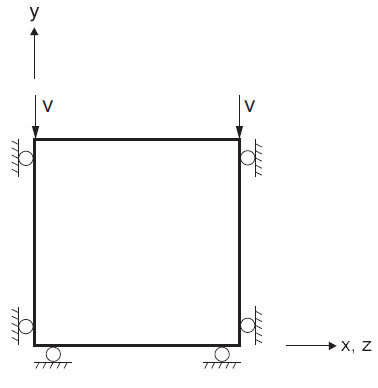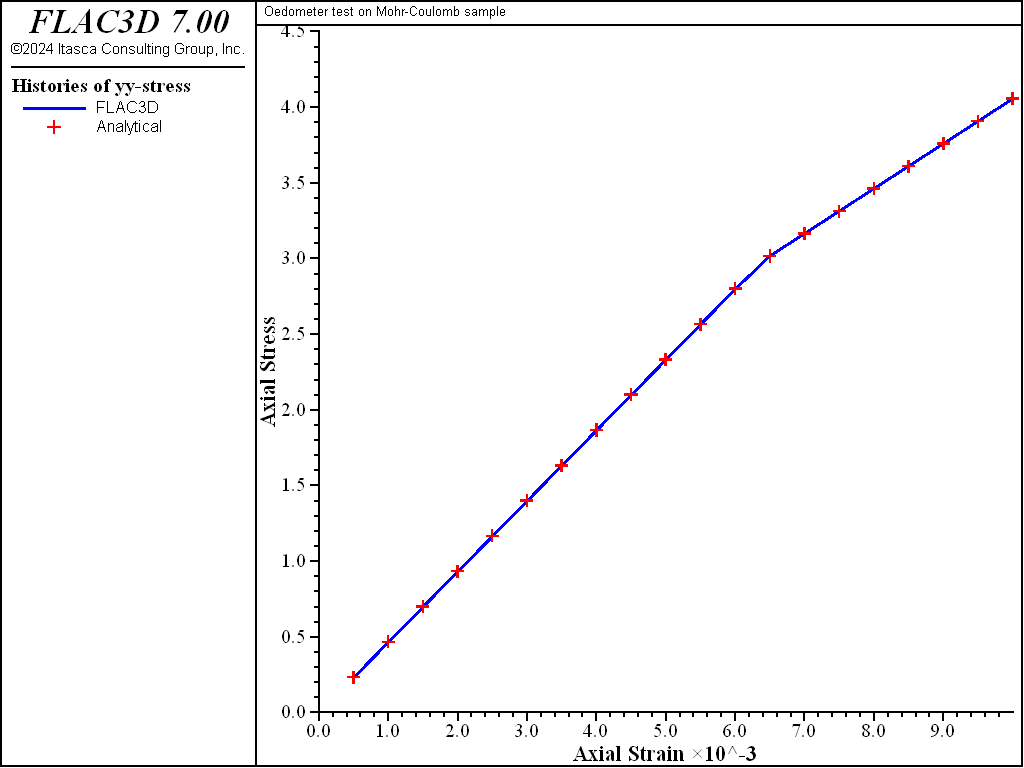FLAC3D Theory and Background • Constitutive Models

# Oedometer Test with Mohr-Coulomb Model

Note

The project file for this example is available to be viewed/run in FLAC3D. The project’s main data file is shown at the end of this example.

This example concerns the determination of stresses in a Mohr-Coulomb material subjected to an oedometer test. In this experiment, two of the principal stress components are equal and, during plastic flow, the stress point evolves along a vertex of the Mohr-Coulomb criterion representation in the $$\Pi$$-plane (the iso-pressure ($$\sigma_{kk}$$ = constant) plane in the principal stress space). The purpose is to validate the numerical technique adopted in FLAC3D to handle such a situation. Note that FLAC3D uses no special techniques to deal with yield at vertex points of the Mohr-Coulomb failure locus in the $$\Pi$$ plane. Results of a numerical experiment are presented and compared to an exact solution.

The boundary conditions for the oedometric test are sketched in Figure 1. They correspond to the uniform strain rates

(1)$\begin{split}\begin{matrix} \Delta {\epsilon}_{11} = 0 \\ \\ \Delta {\epsilon}_{22} = v \Delta t / L \\ \\ \Delta {\epsilon}_{33} = 0 \end{matrix}\end{split}$

where $$v$$ is the constant $$y$$-component of the velocity applied to the sample $$(v < 0)$$, and $$L$$ is the height of the sample.

Assuming zero initial stresses, the principal directions of stresses and strains are those of the coordinate axes. For simplicity, we consider a sample of unit height $$L$$ = 1.Figure 1: Boundary conditions for oedometer test.

In the elastic range, application of Hooke’s law gives, using $${\epsilon}_{22} = v t$$ at time $$t$$:

(2)$\begin{split}\begin{matrix} \sigma_{11} = \alpha_2 v t \\ \\ \sigma_{22} = \alpha_1 v t \\ \\ \sigma_{33} = \sigma_{11} \end{matrix}\end{split}$

where $$\alpha_1 = K + 4/3 G$$ and $$\alpha_2 = K - 2/3 G$$.

To apply the Mohr-Coulomb failure criterion, we consider the yield functions

(3)$\begin{split}\begin{matrix} f^1 = {\sigma}_{22} - {\sigma}_{11} N_{\phi} + 2c \sqrt{N_{\phi}} \\ \\ f^2 = {\sigma}_{22} - {\sigma}_{33} N_{\phi} + 2c \sqrt{N_{\phi}} \\ \end{matrix}\end{split}$

At the onset of yield, $$f^1 = f^2$$ = 0 and, using Equations (2) and (3), we find

(4)$t = {2c \sqrt{N_{\phi}} \over -v(\alpha_1 - \alpha_2 N_{\phi})}$

Hence, yielding will only take place provided $$\alpha_1 - \alpha_2 N_{\phi} >$$ 0.

During plastic flow, the strain increments are composed of elastic and plastic parts, and we have

(5)$\begin{split}\begin{matrix} \Delta {\epsilon}_{11} = \Delta {\epsilon}_{11}^e + \Delta {\epsilon}_{11}^p \\ \\ \Delta {\epsilon}_{22} = \Delta {\epsilon}_{22}^e + \Delta {\epsilon}_{22}^p \\ \\ \Delta {\epsilon}_{33} = \Delta {\epsilon}_{33}^e + \Delta {\epsilon}_{33}^p \end{matrix}\end{split}$

Using the boundary conditions of Equation (1):

(6)$\begin{split}\begin{matrix} \Delta {\epsilon}_{11}^e = - \Delta {\epsilon}_{11}^p \\ \\ \Delta {\epsilon}_{22}^e = v \Delta t - \Delta {\epsilon}_{22}^p \\ \\ \Delta {\epsilon}_{33}^e = - \Delta {\epsilon}_{33}^p \end{matrix}\end{split}$

The flow rule for plastic flow along the edge of the Mohr-Coulomb criterion corresponding to $${\sigma}_{11} = {\sigma}_{33}$$ has the form (e.g., see Drescher 1991)

(7)$\begin{split}\begin{matrix} \Delta {\epsilon}_{11}^p = \lambda_1 {\partial g^1 \over \partial {\sigma}_{11}} + \lambda_2 {\partial g^2 \over \partial {\sigma}_{11}} \\ \\ \Delta {\epsilon}_{22}^p = \lambda_1 {\partial g^1 \over \partial {\sigma}_{22}} + \lambda_2 {\partial g^2 \over \partial {\sigma}_{22}} \\ \\ \Delta {\epsilon}_{33}^p = \lambda_1 {\partial g^1 \over \partial {\sigma}_{33}} + \lambda_2 {\partial g^2 \over \partial {\sigma}_{33}} \end{matrix}\end{split}$

where $$g^1$$ and $$g^2$$ are the potential functions corresponding to $$f^1$$ and $$f^2$$:

(8)$\begin{split}\begin{matrix} g^1 = {\sigma}_{22} - {\sigma}_{11} N_{\psi} \\ \\ g^2 = {\sigma}_{22} - {\sigma}_{33} N_{\psi} \end{matrix}\end{split}$

After partial differentiation, Equation (7) becomes

(9)$\begin{split}\begin{matrix} \Delta {\epsilon}_{11}^p = - \lambda_1 N_{\psi} \\ \\ \Delta {\epsilon}_{22}^p = \lambda_1 + \lambda_2 \\ \\ \Delta {\epsilon}_{33}^p = - \lambda_2 N_{\psi} \end{matrix}\end{split}$

In further considering that by symmetry $$\lambda_1 = \lambda_2$$, we obtain

(10)$\begin{split}\begin{matrix} \Delta {\epsilon}_{11}^p = - \lambda_1 N_{\psi} \\ \\ \Delta {\epsilon}_{22}^p = 2 \lambda_1 \\ \\ \Delta {\epsilon}_{33}^p = - \lambda_1 N_{\psi} \end{matrix}\end{split}$

The stress increments, derived from Hooke’s law, are given by the relations

(11)$\begin{split}\begin{matrix} \Delta {\sigma}_{11} = \alpha_1 \Delta {\epsilon}_{11}^e + \alpha_2 (\Delta {\epsilon}_{22}^e + \Delta {\epsilon}_{11}^e) \\ \\ \Delta {\sigma}_{22} = \alpha_1 \Delta {\epsilon}_{22}^e + \alpha_2 2\Delta {\epsilon}_{11}^e \\ \\ \Delta {\sigma}_{33} = \Delta {\sigma}_{11} \end{matrix}\end{split}$

where we have used the symmetry condition $$\Delta {\epsilon}_{11}^e = \Delta {\epsilon}_{33}^e$$.

Substitution of Equation (6) in Equation (11) yields, using Equation (10):

(12)$\begin{split}\begin{matrix} \Delta {\sigma}_{11} = \alpha_1 \lambda_1 N_{\psi} + \alpha_2 (v \Delta t - 2 \lambda_1 + \lambda_1 N_{\psi}) \\ \\ \Delta {\sigma}_{22} = \alpha_1 (v \Delta t - 2 \lambda_1) + \alpha_2 2 \lambda_1 N_{\psi} \\ \\ \Delta {\sigma}_{33} = \Delta {\sigma}_{11} \end{matrix}\end{split}$

The parameter $$\lambda_1$$ can now be determined by expressing the condition that during plastic flow, $$\Delta f^1$$ = 0. Using Equation (3), this condition takes the form

(13)$\Delta {\sigma}_{22} - \Delta {\sigma}_{11} N_{\phi} = 0$

Substitution of Equation (12) in Equation (13) yields, after some manipulations, the expression

(14)$\lambda_1 = v \Delta t \lambda$

where

(15)$\lambda = {\alpha_1 - \alpha_2 N_{\phi} \over (\alpha_1 + \alpha_2)N_{\phi} N_{\psi} - 2 \alpha_2(N_{\phi} + N_{\psi}) + 2\alpha_1}$

The FLAC3D simulation is carried out using a single zone of unit dimensions. Several properties are used in conjunction with the Mohr-Coulomb model:

 bulk modulus 200 MPa shear modulus 200 MPa cohesion 1 MPa friction 10° dilation 10° and 0° tension 5.67 MPa

The velocity components are fixed in the $$x$$-, $$y$$-, and $$z$$-directions. A velocity of magnitude 10-5 m/steps is applied to the top of the model in the negative $$y$$-direction for a total of 1000 steps. The stress and displacement components in the $$y$$-direction are monitored and compared to the analytic prediction obtained from Equations (2), (4), and (12), using Equations (14) and (15). Two runs are carried out with values of 10° and 0° for the dilation parameter. The match is very good, as can be seen in Figure 2 and Figure 3, where numerical and analytic solutions coincide at the precision of the plot resolution.

breakFigure 2: Oedometric test—comparison of numerical and analytical predictions for 10° dilation.Figure 3: Oedometric test—comparison of numerical and analytical predictions for 0° dilation.

Data File

OedometerMohrCoulomb.dat

;---------------------------------------------------------------------
; oedometer test
; check plastic flow along an edge of the Mohr-Coulomb criterion
;---------------------------------------------------------------------
model new
model large-strain off
fish automatic-create off
zone create brick size 1 1 1
model title "Oedometer test on Mohr-Coulomb sample"
zone cmodel assign mohr-coulomb
zone property bulk 200 shear 200 co 1 friction 10 tension 5.67
[global vyv = -1.e-5]
program call 'oedometerTheoretical'
zone gridpoint fix velocity
zone gridpoint initialize velocity-y [vyv] range position-y 1.0
history interval 50
zone history displacement-y position 0 1 0
fish history n_sy
fish history a_sy
model save 'ini'
; --- dilation 10
zone property dil 10
[d_sigy]
model step 1000
model save 'dil10'
; --- dilation 0
model restore 'ini'
zone property dil 0
[d_sigy]
model step 1000
model save 'dil0'


Endnotes

  To view this project in FLAC3D, use the program menu. Help ▼ Examples…   ⮡   FLAC     ⮡   ConstitutiveModels       ⮡   OedometerMohrCoulomb         ⮡   OedometerMohrCoulomb.prj